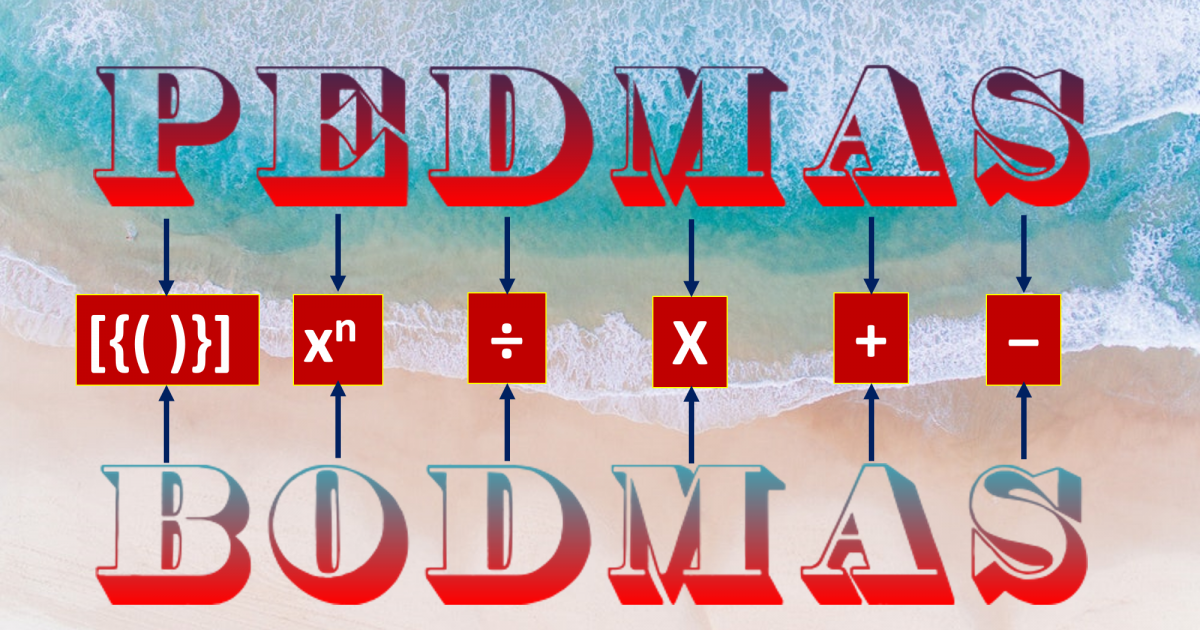Monday, September 27, 2021
Home > CBSE Class 7 > PEMDAS-PEDMAS-BODMAS-BIDMAS-BEDMAS

# PEMDAS-PEDMAS-BODMAS-BIDMAS-BEDMAS# Order of Operations

Hi Students, welcome to Amans Maths Blogs (AMB). In this article, we will study about BODMAS, BIDMAS, BEDMAS, PEDMAS or PEMDAS. We will also learn about how to use these in simplification of expressions. These mathematical terms are in the syllabus of CBSE Class 7 Maths of chapter INTEGERS.

In the chapter Integers of Class 7, we learnt about the integers, addition of integers, subtraction of integers, multiplication of integers, division of integers and their properties like closure, commutative, associative, additive identity, multiplicative identity etc.

Therefore, we can solve some simple maths questions of addition, subtraction, multiplication and division of integers like 12 + 6, (-35) x 5, 12 + 6 + 9, 25 – 2 etc.

In these types of maths problems, there is one and only one mathematical operator between the operands (In 12 + 6; plus (+) sign is known as mathematical operator whereas 12 and 6 are known as operands) at a time.

Now, suppose there are more than one mathematical operator between the operands.

For examples: 12 + 6 x 10 or 2 – (12 x 3 + 5 – 6) etc.

How to simplify it and how to find its answer?  What are the order of operations to be performed first; first we add or first we multiply or subtract?

To solve these types of maths problems, the order of operators are defined, which is known as BODMAS or BEDMAS or BIDMAS or PEMDAS or PEDMAS.

## BODMAS or BEDMAS or BIDMAS

The BODMAS is a concept that help us to simplify the math problems containing more than one mathematical operators.

BODMAS is an acronym and it full form is as below.

 B → Brackets ( ), { }, [ ] O → Order (Exponent or Index) D → Division (÷) M → Multiplication (x) A → Addition (+) S → Subtraction (-)

Thus, according to BODMAS rule,

First, we solve the brackets (B) and the order of brackets is as Small ( ), Curly or Middle { } and at last Square or Big [ ], if the math question contains.

And then, we solve the order (O). The order means exponent or index. Therefore, BODMAS rule is also known as BEDMAS (in this, E means Exponents) or BIDMAS (in this, I means Index). It means, we solve the exponent if the math problem contains.

And then, we solve the division (D)if the math question contains.

And then, we solve the multiplication (M)if the math question contains.

And then, we solve the addition (A)if the math question contains.

And then, we solve the subtraction (S)if the math question contains.

For example: we need to solve, 12 + [5 x {24 ÷ 2 +  (9 ÷ 3)}].

This question contains brackets, division, multiplication, addition and subtraction. It does not contain order or exponents. We can ignore the operation of order (O) in BODMAS.

Thus, using BODMAS rule, we get the solution of the given question as below:

12 + [5 x {24 ÷ 2 +  (9 ÷ 3)}]
= 12 + [5 x {24 ÷ 2 +  (9 ÷ 3)}]   Performing Small Bracket ( )
= 12 + [5 x {24 ÷ 2 +  3}]
= 12 + [5 x {24 ÷ 2 +  3}]           Performing Curly Bracket { }
= 12 + [5 x {12 +  3}]
= 12 + [5 x 15]
= 12 + [5 x 15]                          Performing Square Bracket [ ]
= 12 + 75 = 87                          Performing Addition (+)

## PEDMAS or PEMDAS

In India, the simplification or order of mathematical operations is knwon as BODMAS.

But, in some country like US (United States) or UK (United Kingdom) etc, the BODMAS rule is known as PEDMAS.

The PEDMAS is a concept that help us to simplify the math problems containing more than one mathematical operators.

PEDMAS is an acronym and it full form is as below.

 P → Parentheses ( ), { }, [ ] E → Exponent or Index D → Division (÷) M → Multiplication (x) A → Addition (+) S → Subtraction (-)

Now, the orders of division and multiplication in PEDMAS and PEMDAS are different.

Since the multiplication and division are the same priority when solving problems involving multiplication/division, then the rule to remember is to use the operation multiplication/division in the order they appear when going from left to right in the equation.

For example: the expression 12 x 6 ÷ 3 (from left to right: multiplication comes before division) is simplified by using PEMDAS and the expression 12 ÷ 6 x 3 (from left to right: division comes before multiplication) is simplified by using PEDMAS

Thus, we can say that:

BODMAS, BEDMAS, BIDMAS, PEDMAS and PEMDAS, all are same.

Get Online Maths Tuition by AMBiPi for Class 8 to 12